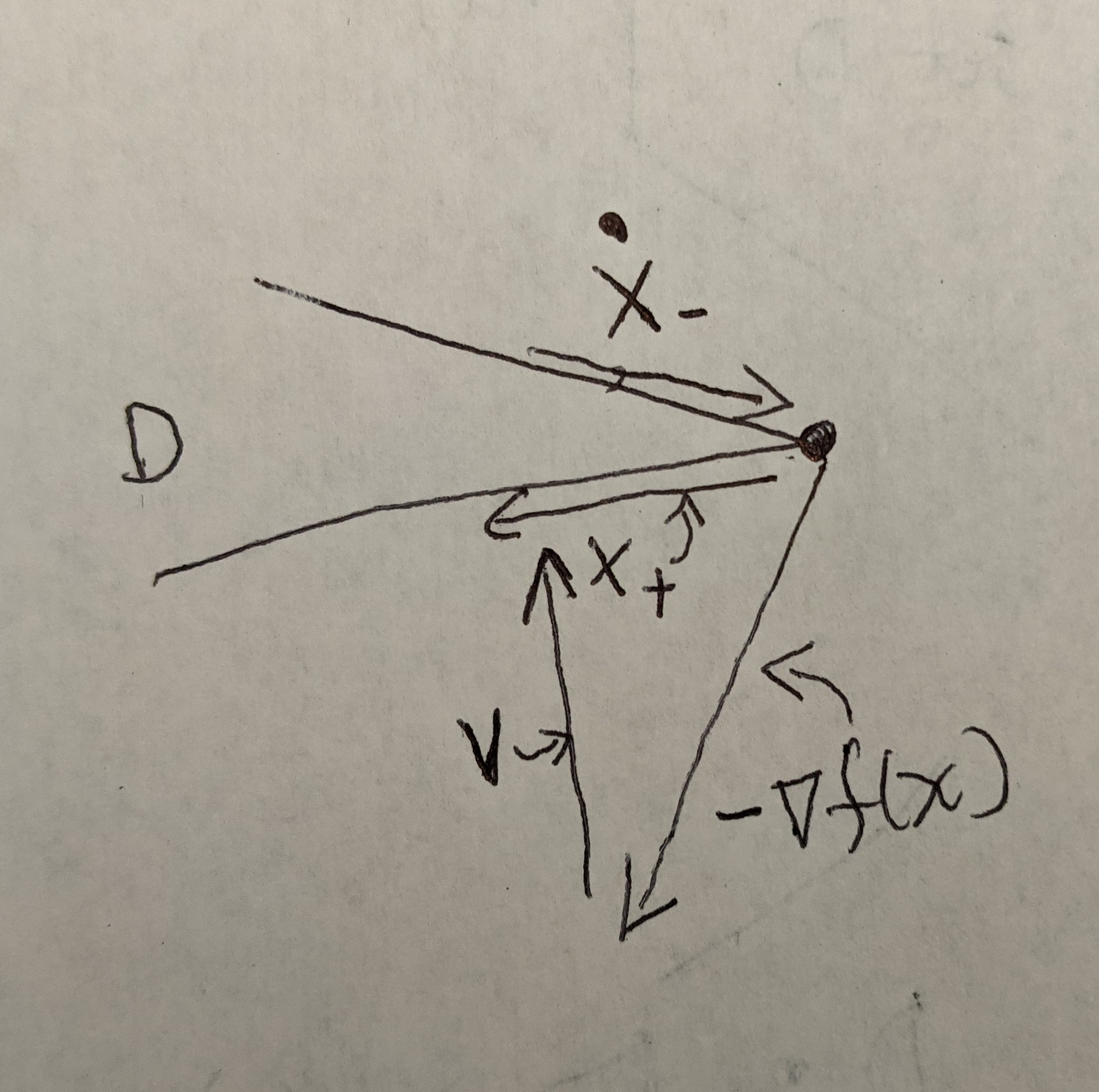# Some proofs of continuous time things

In trying to understand how continuous time interpretations can help optimization research, I find myself doing a lot of “recreating the wheel”, but in a nontrivial way; I am getting these proofs that I’m sure are standard knowledge, but it’s not like we learned them back when we were kids. So I’m going to try and go through some of them here.

Here are some super simple proofs to get us started.

This one is actually super easy and classical, with one of the earliest citations . Here, we’re looking at unconstrained methods

$$\min_{x} \quad f(x)\tag{(U)}$$

and the method is very simply

\begin{eqnarray*}
\mathbf x_{k+1} &=& \mathbf x_k   – \alpha \nabla f(\mathbf x_k)
\end{eqnarray*}

with flow

\begin{eqnarray*}
\dot x(t) &=& -\nabla f(x(t)).
\end{eqnarray*}

Super classical. Then the convergence proof of the flow is simply

$$\frac{d}{dt} f(x(t))= \nabla f(x)^T\dot x = -\|\nabla f(x)\|_2^2.$$

Right away, we get method descending, and (after showing that $\|\nabla f(x)\|_2$ also decays)  a rate

$$\|\nabla f(x(t))\|_2^2 \leq \frac{1}{t} \int_0^t\|\nabla f(x(\tau))\|_2^2d\tau \leq \frac{f(x(0))-f(x^*)}{t}$$

I’ve already discussed this one a bit in a previous post, so I’ll just stop here. Note that I did not say anything about convexity, compactness, etc.

Now let’s make the problem slightly more complicated by doing the projected version.  Here, we want to solve

$$\min_{x\in\mathcal D} \quad f(x)\tag{C}$$

where $\mathcal D\subset \mathbb R^n$ is a convex, closed set.

We use the repeated iteration

\begin{eqnarray*}
\mathbf x_{k+1} &=& \mathrm{proj}_{ \alpha g}(\mathbf x_k   – \alpha \nabla f(\mathbf x_k))\tag{PGD}
\end{eqnarray*}

where

$$\mathrm{proj}_{g}(z):= \arg\min_{x\in \mathcal D} \; \|x-z\|_2.$$

One way to reframe the method, then, is iteratively solving the following problem at each step

\begin{eqnarray*}
\mathbf x_{k+1} &=& \arg\min_x \frac{1}{2}\|x – \mathbf x_k   + \alpha \nabla f(\mathbf x_k)\|_2^2 + I_{\mathcal D}(x)
\end{eqnarray*}

where $I_{\mathcal D}(x)$ is the indicator for the constraint $x\in \mathcal D$.

From that, we can write the optimality condition

$$\mathbf x_{k} – \mathbf x_{k+1} \in \alpha \nabla f(\mathbf x_k) + \mathcal N_{\mathcal D}(\mathbf x_{k+1}) \tag{PGD-opt}$$

and $\mathcal N_{\mathcal D}(x)$ is the normal cone of $\mathcal D$ at $x$, described as

$$\mathcal N_{\mathcal D}(x) := \partial I_{\mathcal D}(x) = \{z : z^T(x-x’) \geq 0, \; \forall x’\in \mathcal D\}. \tag{NC-def}$$

And, again using approximations of $\dot x(t) \approx \frac{\mathbf x_{k+1} – \mathbf x_{k}}{\alpha}$ we get

$$-\dot x – \nabla f(x) \in \mathcal N_{\mathcal D}(x). \tag{PGD-flow}$$

(I’m  a bit skeptical to do this, because then it seems this method is the same as the subgradient method, which I kind of assumed would have a worse rate. But, then again… maybe not in the flow? After all, what is the harm of nonsmoothness in the continuous time regime? Still, I’d feel more comfortable if some $\frac{d}{dt} \nabla f(x)$ term appeared somewhere. For now we’ll go with it.)

Now here comes a crucial step. In the usual proof of the projected gradient method, we needed to show that projections cannot increase your distance to $x^*$. I wrote this as a “funny little proof” in a previous post. But in the continuous version, what we really want to say is that projections can only slow you down in your desired direction–that is, the $\dot x$ from PGD can’t be faster than the $\dot x$ from good ole GD. Concretely, I want to say that the $\dot x$ defined in (PGD-flow) satisfies

$$\|\dot x\|_2 \leq \|\nabla f(x)\|_2. \tag{Proj-Contr-Cond.}$$

This is going to work as follows. We define $v(t) = -\dot x(t) – \nabla f(x(t))$. Then by (PGD-Flow), $v\in \mathcal N_\mathcal D(x)$, or $v^T(x-x’) \geq 0$, for all $x’\in \mathcal D$. I’m going to pick $x’ = x – c \dot x$, for some imperceptibly small $c > 0$. Note that since the trajectory is always feasible, such a $c>0$ should exist, if we assume we initialize the method somewhere in $\mathcal D$.  Then, I can say that $v^T\dot x \geq 0$.

Then, using Pythagorean theorem,

$$\|\nabla f(x)\|_2^2 = \|v+\dot x\|_2^2 = \|v\|_2^2 +\underbrace{ 2v^T\dot x}_{\geq 0} + \|\dot x\|_2^2 \geq \|\dot x\|_2^2 \tag{Proj-Contr-Cont}$$

And we’re done! See below for the super-high-tech figure.Projected gradient descent. Note the almost Pythagorean relationship between $\dot x$, $v$, and $\nabla f(x)$.

Ok, Now we can move onto the main event: convergence proof!

$$\frac{d}{dt} f(x(t)) = \nabla f(x)^T\dot x \leq (\nabla f(x) + v)^T{\dot x} = -\|\dot x\|_2^2$$

and therefore

$$\frac{f(0)-f(x^*) }{t}\geq \frac{1}{t}\int_0^t \|\dot x(\tau)\|_2^2 d\tau$$

e.g. we have an $O(1/t)$ bound on the average speed of the method. Note that it makes perfect sense why we can’t seem to be able to bound the average gradient of the method, since in a constrained problem, $\nabla f(x(t))\not\to 0$.

The proof is more -or-less trivially extended to the proximal case, e.g. we solve

$$\min_{x} \quad f(x) + g(x)\tag{(U2)}$$

using the repeated iteration

$$\mathbf x_{k+1} = \mathrm{prox}_{ \alpha g}(\mathbf x_k – \alpha \nabla f(\mathbf x_k)), \qquad \mathrm{prox}_{g}(z):= \arg\min_x \; g(x) + \frac{1}{2}\|x-z\|_2^2.$$

Edit: It occurred to me that a very strange thing happens when $x(t)$ is on a corner of $\mathcal D$, which brings out a problem with nonsmoothness. In this case, $\dot x(t)$ is not really well defined: we have

$$\dot x_+(t) = \lim_{\Delta>0,\Delta \to 0} \frac{x(t+\Delta)-x(t)}{\Delta}, \qquad \dot x_-(t) = \lim_{\Delta>0,\Delta \to 0} \frac{x(t)-x(t-\Delta)}{\Delta}$$A literal “corner” case, showing that it matters if the velocity is coming from the future or the past.

and they’re both different. Note that our relationship (Proj-Contr-Cont) only applies to $\dot x_+$, not $\dot x_-$ in this case. See image above.

 Polyak, Boris T. “Introduction to optimization. 1987.” Optimization Software, Inc, New York.